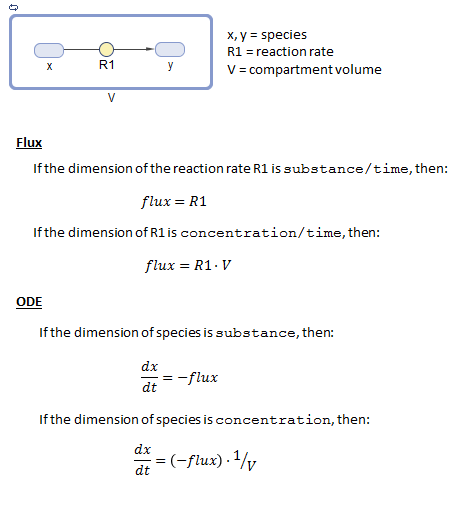Main Content

## Deriving ODEs from Reactions

For model simulation, SimBiology® derives ordinary differential equations (ODEs) from model reactions using mass-balance principles. The left-hand-side (LHS) of each ODE is the time-derivative of a model quantity and the right-hand-side (RHS) is defined using reaction fluxes that are derived from reaction rates and rate rules. In other words, SimBiology represents a system of ODEs as:

$\stackrel{˙}{x}=S\cdot v$

$\stackrel{˙}{x}$ is an M-by-`1` vector containing the rates of change for model quantities, S is an M-by-R stoichiometry matrix, v is an R-by-`1` flux vector. `M` equals the total number of species, and `R` equals the total number of reactions in the model

During the conversion of model reactions into ODEs, SimBiology performs a dimensional analysis to ensure each reaction flux has the dimension of `substance/time` such as `amount/time` or `mass/time`. If the reaction rate has the dimension of `concentration/time`, then SimBiology multiplies it by the compartment volume to get the reaction flux. If the reaction rate has the dimension of `substance/time`, then the flux is identical to the rate, and no volume-correction is performed. If there are no units specified with the model, the default dimension for a species (`DefaultSpeciesDimension`) is `concentration`, and that for a flux is `substance/time`. For such cases, the ODE is the flux divided by a compartment volume to make the dimension of LHS and RHS consistent. See the following figure for an illustration.

Suppose there is a reaction `x —> y`, with the reaction rate R1. The following figure explains the dimensional analysis performed by SimBiology to make the dimensions of LHS and RHS of an ODE consistent.## Support평가판 신청